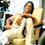# E-L-L-I-P-S-E

How to find the perimeter of an ellipse???Note by Anirudha Nayak
7 years, 3 months ago

This discussion board is a place to discuss our Daily Challenges and the math and science related to those challenges. Explanations are more than just a solution — they should explain the steps and thinking strategies that you used to obtain the solution. Comments should further the discussion of math and science.

When posting on Brilliant:

• Use the emojis to react to an explanation, whether you're congratulating a job well done , or just really confused .
• Ask specific questions about the challenge or the steps in somebody's explanation. Well-posed questions can add a lot to the discussion, but posting "I don't understand!" doesn't help anyone.
• Try to contribute something new to the discussion, whether it is an extension, generalization or other idea related to the challenge.

MarkdownAppears as
*italics* or _italics_ italics
**bold** or __bold__ bold
- bulleted- list
• bulleted
• list
1. numbered2. list
1. numbered
2. list
Note: you must add a full line of space before and after lists for them to show up correctly
paragraph 1paragraph 2

paragraph 1

paragraph 2

[example link](https://brilliant.org)example link
> This is a quote
This is a quote
    # I indented these lines
# 4 spaces, and now they show
# up as a code block.

print "hello world"
# I indented these lines
# 4 spaces, and now they show
# up as a code block.

print "hello world"
MathAppears as
Remember to wrap math in $$ ... $$ or $ ... $ to ensure proper formatting.
2 \times 3 $2 \times 3$
2^{34} $2^{34}$
a_{i-1} $a_{i-1}$
\frac{2}{3} $\frac{2}{3}$
\sqrt{2} $\sqrt{2}$
\sum_{i=1}^3 $\sum_{i=1}^3$
\sin \theta $\sin \theta$
\boxed{123} $\boxed{123}$

Sort by:

Let the ellipse be : $\displaystyle \frac{x^2}{a^2}+\frac{y^2}{b^2} = 1$

Parametric coordinates : $\displaystyle (x,y) = (a\cos\theta,b\sin\theta)$

$ds^2 = dx^2+dy^2$

$ds^2 = \left((\frac{dx}{d\theta})^2 + (\frac{dy}{d\theta})^2\right)d\theta^2$

$ds^2 = (a^2\sin^2\theta+ b^2\cos^2\theta)d\theta^2$

$ds = \sqrt{(a^2\sin^2\theta+ b^2\cos^2\theta)}d\theta$

Integrate it from $\displaystyle 0$ to $\displaystyle 2\pi$, and you will get the result.

- 7 years, 3 months ago

Good luck integrating that monster.! (it hasn't been solved yet)

- 7 years, 3 months ago

i am surprised This question came in VIT couldnt solve it maths was at a good level indeed when is urs or have u already given it

- 7 years, 3 months ago

It was yesterday. What was the exact question?

- 7 years, 3 months ago

i have posted it

- 7 years, 3 months ago

how was d exam wht r u expecting

- 7 years, 3 months ago

atleast 100 to aana chahiye.

- 7 years, 3 months ago

ans for d question

- 7 years, 3 months ago

me tooo simple paper though

- 7 years, 3 months ago

There is no exact formula but use the famous Indian mathematician Ramanujan came up with this better approximation:search Ramanujan circumference of ellipse formula

- 7 years, 3 months ago

Were there any options? I am asking because as Anish said that the value of the integral has no clear form. Maybe they intended to ask something different??

- 7 years, 3 months ago

$\pi(2^{1/2}),2\pi(2^{1/2}),\pi(2+2^{1/2})$ and one other i dont remember them clearly

- 7 years, 3 months ago